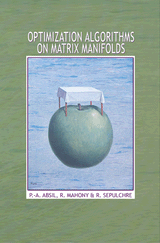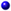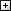Processing ......FreeComputerBooks.com Free Computer, Mathematics, Technical Books and Lecture Notes, etc.

Optimization Algorithms on Matrix Manifolds
For weather and flight schedules of Airports all over the world, click Here.
• Title Optimization Algorithms on Matrix Manifolds
• Authors P.-A. Absil, R. Mahony, R. Sepulchre
• Publisher: Princeton University Press; illustrated edition edition (December 3, 2008)
• Paperback: 240 pages
• Language: English
• ISBN-10: 0691132984
• ISBN-13: 978-0691132983Book Description

Many problems in the sciences and engineering can be rephrased as optimization problems on matrix search spaces endowed with a so-called manifold structure. This book shows how to exploit the special structure of such problems to develop efficient numerical algorithms. It places careful emphasis on both the numerical formulation of the algorithm and its differential geometric abstraction--illustrating how good algorithms draw equally from the insights of differential geometry, optimization, and numerical analysis. Two more theoretical chapters provide readers with the background in differential geometry necessary to algorithmic development. In the other chapters, several well-known optimization methods such as steepest descent and conjugate gradients are generalized to abstract manifolds. The book provides a generic development of each of these methods, building upon the material of the geometric chapters. It then guides readers through the calculations that turn these geometrically formulated methods into concrete numerical algorithms. The state-of-the-art algorithms given as examples are competitive with the best existing algorithms for a selection of eigenspace problems in numerical linear algebra.

Optimization Algorithms on Matrix Manifolds offers techniques with broad applications in linear algebra, signal processing, data mining, computer vision, and statistical analysis. It can serve as a graduate-level textbook and will be of interest to applied mathematicians, engineers, and computer scientists.

The treatment strikes an appropriate balance between mathematical, numerical, and algorithmic points of view. The quality of the writing is quite high and very readable. The topic is very timely and is certainly of interest to myself and my students."--Kyle A. Gallivan, Florida State UniversityAll CategoriesRecent BooksIT Research LibraryMiscellaneous BooksComputer LanguagesComputer ScienceData Science/DatabasesElectronic EngineeringJava and Java EE (J2EE)Linux and UnixMathematicsMicrosoft and .NETMobile ComputingNetworking and CommunicationsSoftware EngineeringSpecial TopicsWeb Programming